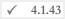# #mathContextUsing the #mathContext system object, the calculation accuracy and rounding mode can be configured for the division of numbers (e.g. \$CMS_VALUE(100.0/33.0)\$).

The system object features the precision, roundingMode and useDefault methods.

The division accuracy can be influenced using the precision method. An integer can be entered as the value which specifies the number of decimal places for the calculation. The default value is 32.

Example:

`\$CMS_SET(#mathContext.precision, 3)\$`

The number of decimal places is set to 3 for the calculation using this \$CMS_SET(...)\$ instruction.

The result of \$CMS_VALUE(100.0/33.0)\$ would thus be

`3.03`

The rounding mode can be specified using the roundingMode method. The following are possible values:

• UP
• DOWN
• CEILING
• FLOOR
• HALF_UP
• HALF_DOWN
• HALF_EVEN and
• UNNECESSARY

HALF_UP is used as the default value.
The description of individual rounding modes can be found in the general Java API documentation:
RoundingMode

Example:

`\$CMS_SET(#mathContext.roundingMode, "UP")\$`

Using this \$CMS_SET(...)\$ instruction, the rounding mode is set to the value UP.

The result of \$CMS_VALUE(100.0/33.0)\$ would thus be

`3.0303030303030303030303030303031`

The calculation accuracy and rounding mode can be reset to the default values using the useDefault method:

`\$CMS_SET(_void, #mathContext.useDefault)\$`